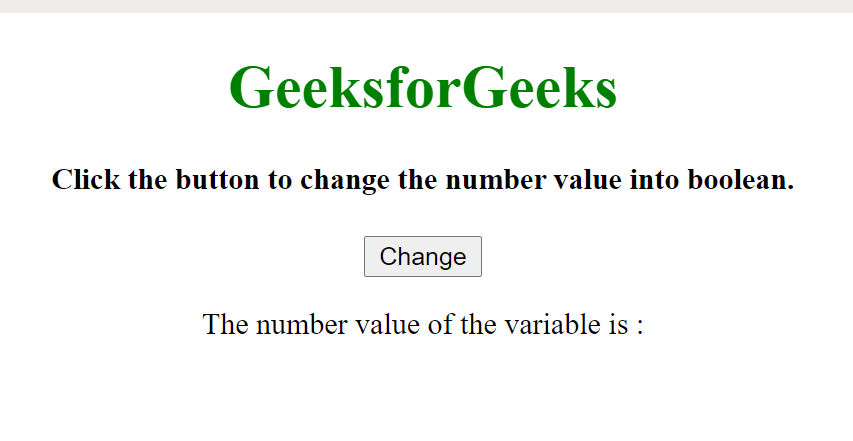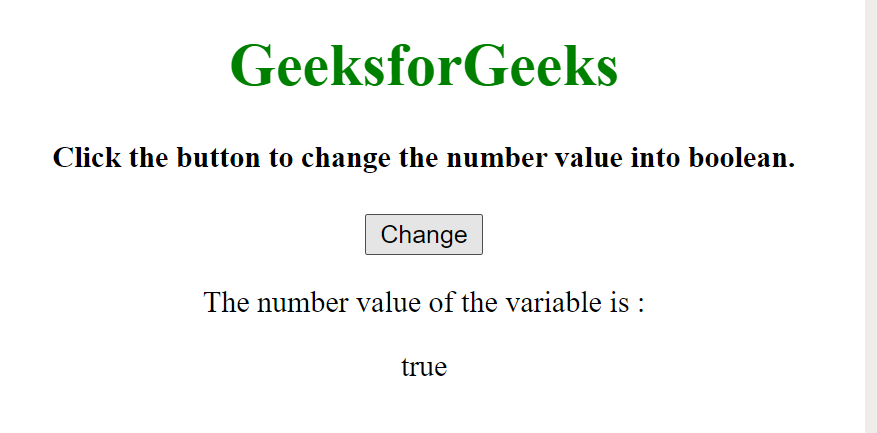# How to convert Number to Boolean in JavaScript ?

• Last Updated : 08 Jan, 2021

We convert a Number to Boolean by using the JavaScript Boolean() method. A JavaScript boolean results in one of the two values i.e true or false. However, if one wants to convert a variable that stores integer “0” or “1” into Boolean Value i.e “true” or “false”.

Syntax:

`Boolean(variable/expression)`

Example:

## HTML

 ` ` `<``html``> ` ` `  `<``body``> ` `    ``<``center``> ` `        ``<``h1` `style``=``"color:green"``> ` `            ``GeeksforGeeks ` `        `` ` ` `  `        ``<``h4``> ` `            ``Click the button to change the ` `            ``number value into boolean. ` `        `` ` ` `  `        ``<``button` `onclick``=``"myFunction()"``>Change ` ` `  `        ``<``p``>The number value of the variable is : ` ` `  `        ``<``p` `id``=``"result"``> ` ` `  `        ``<``script``> ` ` `  `            ``// Initializing boolvalue as true  ` `            ``var numvalue = 1; ` ` `  `            ``// JavaScript program to illustrate boolean  ` `            ``// conversion using ternary operator  ` `            ``function myFunction() { ` ` `  `                ``document.getElementById("result") ` `                    ``.innerHTML = Boolean(numvalue); ` `            ``}  ` `        `` ` `    `` ` ` ` ` `  ` `

Note: If the above Boolean() method is called as Boolean(!numvalue), it shows the result as “false“. Similarly if it is called as Boolean(!!numvalue), it gives the result as “true“.

Output:

Before clicking on button:After clicking on button:My Personal Notes arrow_drop_up
Recommended Articles
Page :